# Electric Power and Electric Energy

(This article was translated from Japanese to English using DeepL.)

This is the final lecture of Electromagnetism in Bacic Physics. The last theme is about electric power and electric energy.

## Work performed by the electric current

Physics textbooks state that “Electric power is the work done by an electric current”.

Of course, this statement is correct. But do you really understand it from this description alone?

So I would like to elaborate a little more.　First of all, the “work” in this text is the same concept as the work we learned about in mechanics.

https://www.yukimura-physics.com/entry/dyn-f23

“Work = Moving an object by force”, but there was one more important point. It is work as a variant of energy.

Let’s review briefly. Suppose an object with a potential energy of 100 J falls and its potential energy is reduced to 20 J.
What happens to the reduced energy (80 J) is that it becomes kinetic energy of the object.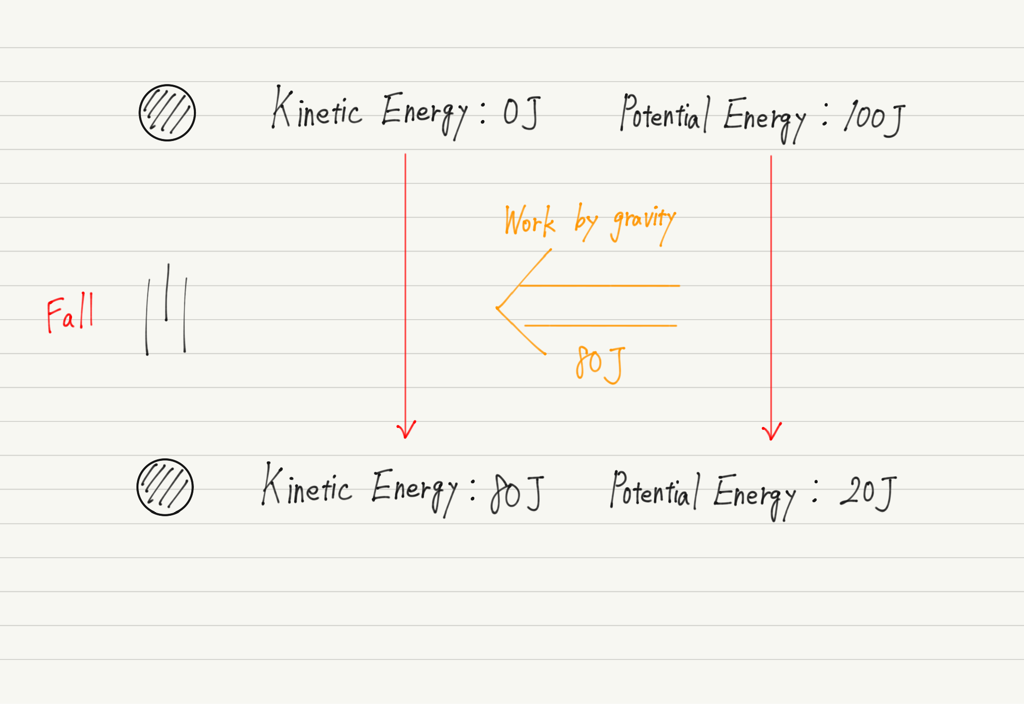In other words,
The potential energy due to gravity is reduced.
↓
Gravity does work on the object for the reduced potential energy.
↓
The kinetic energy of an object increases with the amount of work done.

The key point is the conversion of potential energy into kinetic energy via work.
The work that electric current does can be understood in this same way.

This is because, as we discussed in the Ohm’s law lecture, the phenomenon of “Electric current passing through a resistance” is the same as “Falling from a high voltage to a low voltage”!

https://www.yukimura-physics.com/entry/elemag-f06

In the lecture on voltage, we described “Voltage is electrical height”, but more precisely, “Voltage is electrical potential energy”.

Positive side of battery　→　Large potential energy

Negative side of battery　→　Small potential energy

Since electric current always flows from the positive toward the negative, the potential energy of the electricity is reduced as the current flows through the resistance!

Consider this in comparison with the previous example of an object falling. In the fall of an object, work was done on the object for the reduced potential energy. The same thing naturally happens even with electricity.

The reduced electrical potential energy generates work! This is what “the work done by an electric current” is all about!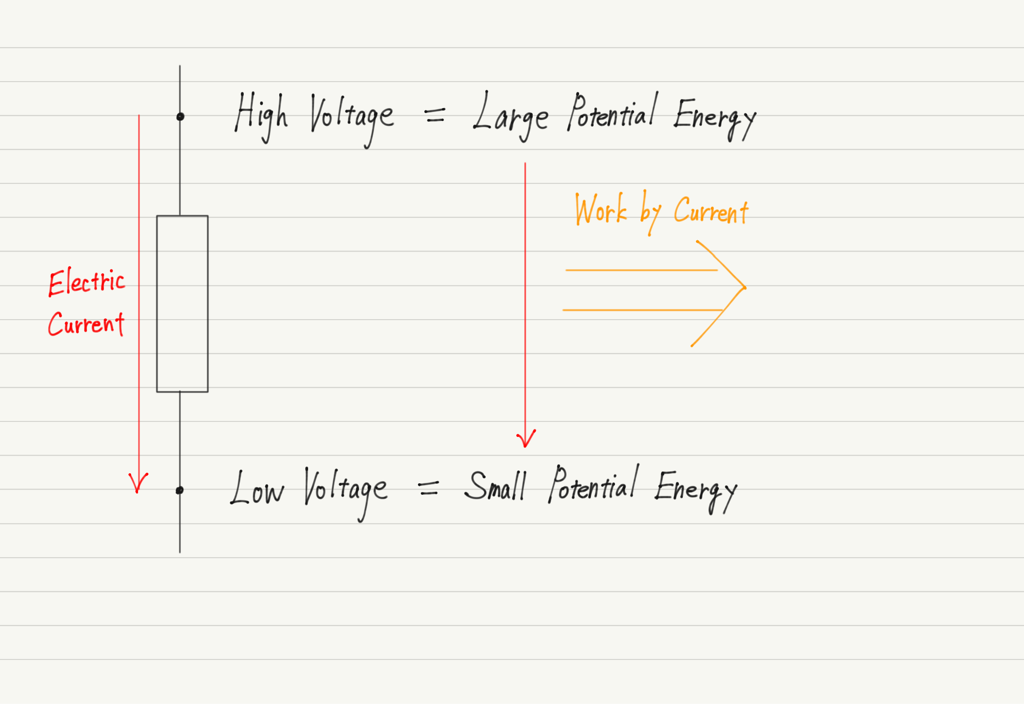## Work done by electric current and Joule heat

By the way, what is the object on which the electric current does its work?

The answer to this question has already been done in the previous lecture.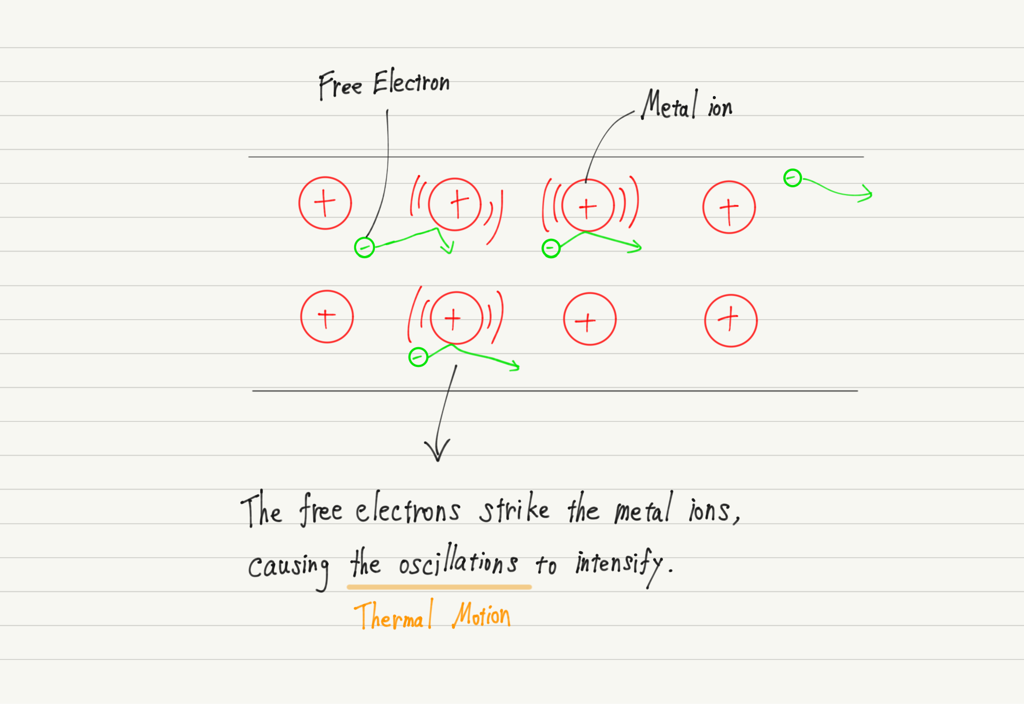（The figure is reproduced.）

The current does work on the metal ions that make up the resistance, as shown in this figure!

However, metal ions are not free to move, so they oscillate (Thermal motion) in place.
To summarize so far,
Electric current flows through a resistance (from high voltage to low voltage).
↓
Decreased electrical potential energy.
↓
The current does work on the metal ions of the resistor to the extent that the potential energy is reduced.
↓
The more work done, the more energy for thermal movement.
↓
Joule heat is generated.

## Calculation of Electric Power and Electric Energy

Let’s get our story straight. We now know that the electric energy is the work done by the electric current and that this is related to Joule heat.

Now, when an object falls, its kinetic energy increases by the amount of work done by gravity.
The same is true for electricity, where the energy of thermal motion is increased by the work done by the electric current.
That means the electric energy is exactly equal to the Joule heat generated!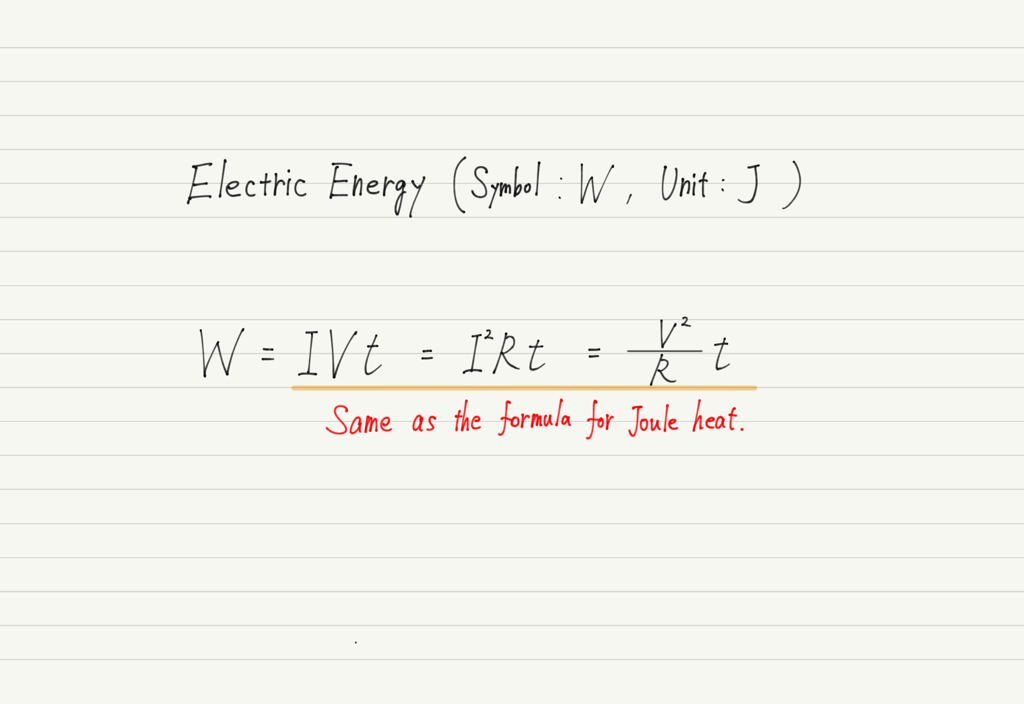If electric energy is the work done by the electric current, then there is also the concept of the “Power” done by the current. That is electric power.
The unit of electric energy is J and the unit of electric power is W. Be careful not to mix them up.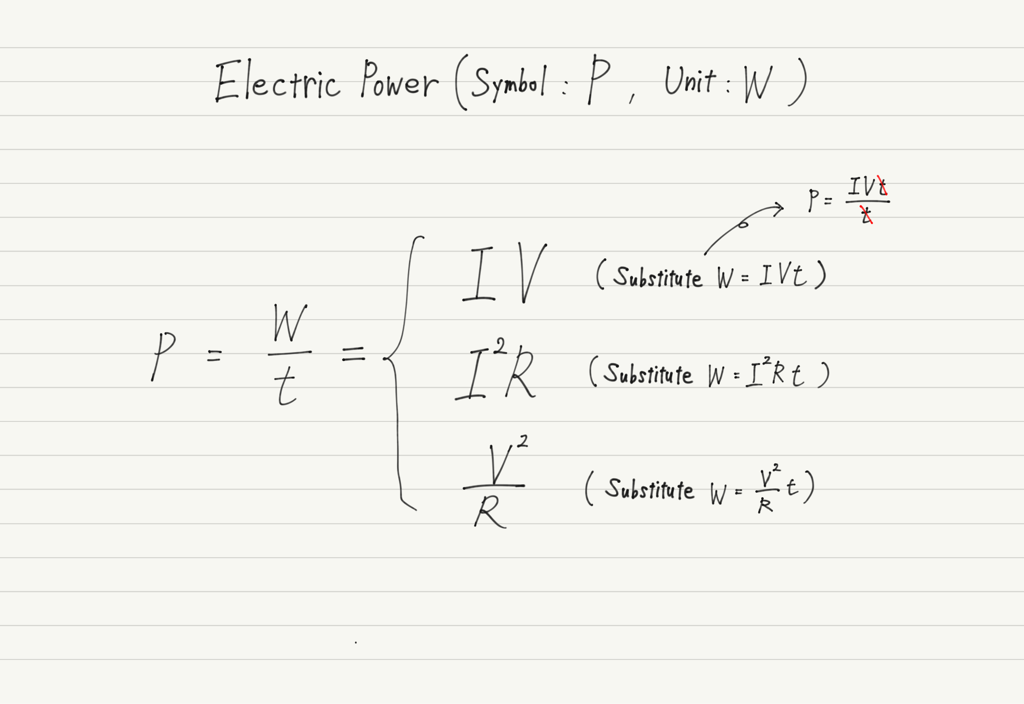## Summary of this lecture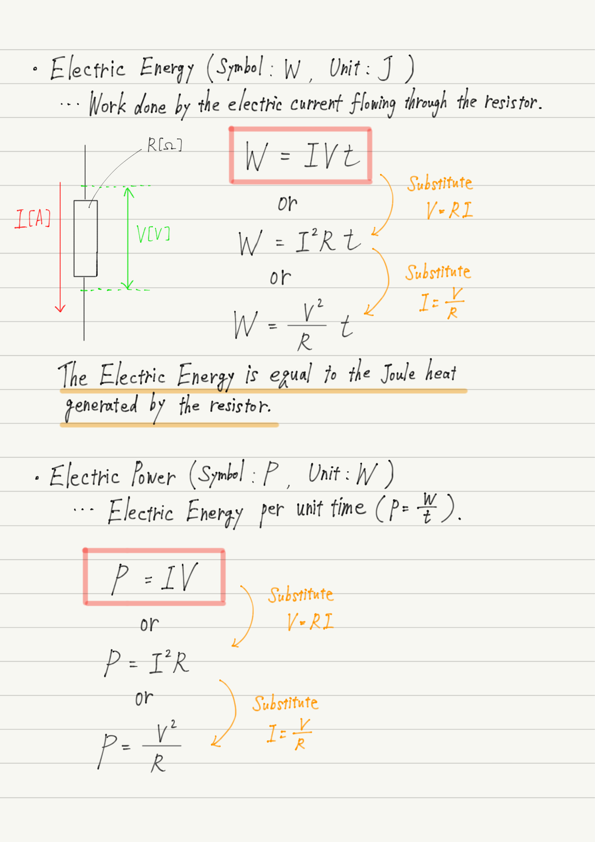## Next Time

This concludes electromagnetism in Basic Physics.
After this, we will move on to Advanced Physics, which is more difficult than Basic Physics.
In addition, some of them require knowledge of mechanics, so please complete all areas of Basic Physics (including Appendix) before proceeding.

https://www.yukimura-physics.com/entry/elemag01

error: Content is protected !!